# Samacheer Kalvi Books: Tamilnadu State Board Text Books Solutions

## Wednesday, January 5, 2022Samacheer Kalvi 7th Maths Algebra Notes PDF Download: Tamil Nadu STD 7th Maths Algebra Notes

We bring to you specially curated Samacheer Kalvi 7th Maths Algebra Notes PDF which have been prepared by our subject experts after carefully following the trend of the exam in the last few years. The notes will not only serve for revision purposes, but also will have several cuts and easy methods to go about a difficult problem.

 Board Tamilnadu Board Study Material Notes Class Samacheer Kalvi 7th Maths Subject 7th Maths Chapter Algebra Format PDF Provider Samacheer Kalvi Books

2. Click on the Samacheer Kalvi 7th Maths Notes PDF.
3. Look for your preferred subject.

## Tamilnadu Samacheer Kalvi 7th Maths Solutions Term 1 Chapter 3 Algebra Intext Questions

Exercise 3.1

Try These (Text Book Page No. 51)

Question 1.
Identify the variable and constants among the following terms.
a, 11 – 3x, xy, -89, -m, -n, 5, 5ab, -5 3y, 8pqr, 18, -9t, -1, -8
Solution:
Variable : a, -3x, xy, -m, -n, 5ab, 3y, -9t, 8pqr
Constants : 11, -89, 5, -5, 18, -1, -8

Question 2.
Complete the following table.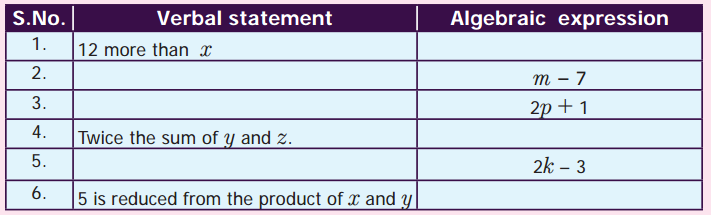Solution: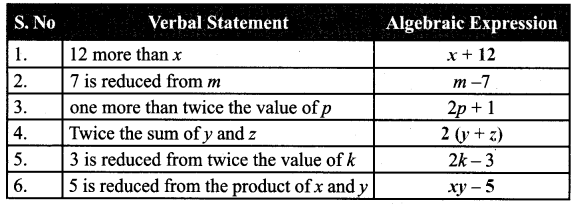Try this (Text book Page No. 53)

Question 1.
Can we use the operations multiplication and division to combine terms?
Solution:
No, We can use addition and subtraction to combine terms.
If we use multiplication or division to combine then it become a single term.
Eg : xy, xy are monomials.

Try This (Text book Page No. 54)

Question 1.
Complete the following table by forming expressions using the terms given. One is done for you.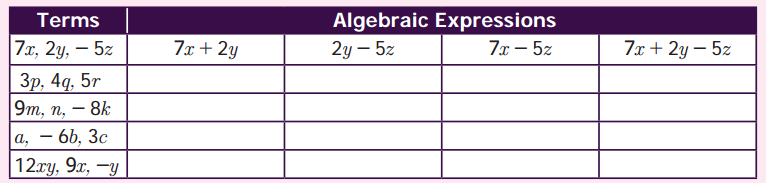Solution: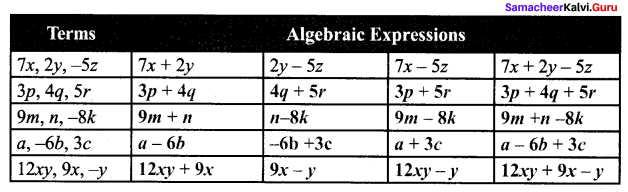Try this (Text book Page No. 56)

Question 1.
Identify the like terms among the following and group them.
7xy, 19x, 1, 5y, x, 3yx, 15, -13y, 6x, 12xy, -5, 16y, -9x, 15xy, 23, 45y, -8y, 23x, -y, 11
Solution:
7xy, 3yx, 12xy, 15xy, are like terms
19x, x, 6x,-9x, 23x, are like terms
5y, -13y, 16y, 45y, -8y, -y, are like terms
1, 15, -5, 23, 11, are like terms.

Try This (Text book Page No. 57)

Question 1.
Try to find the value of the following expressions if p = 5 and q = 6.
(i) p + q
(ii) q – p
(iii) 2p + 2 > q
(iv) pq – p – q
(v) 5pq – 1
Solution:
(i) Given p = 5; q = 6
p + q = 5 + 6 = 11
(ii) q – p = 6 – 5 = 1
(iii) 2p + 2 > q = 2(5) + 3(6) = 10 + 18 = 28
(iv) pq – p – q = (5) (6) – 5 – 6 = 30 – 5 – 6 = 25 – 6 = 19

Exercise 3.2

Try These (Text book Page No. 59)

Question 1.
(i) 3p, 14p
(ii) m, 12m, 21m
(iii) 11abc, 5abc
(iv) 12y, -y
(v) 4x, 2x, -7x.
Solution:
(i) 3p + 14p = 17p
(ii) m + 12m + 21m = (1 + 12 + 21 )m
= 34 m
(iii) 11abc + 5abc = (11 + 5) abc
= 16 abc
(iv) 12y + (-y) = (12 + (-1))y
= (12 – 1 )y
= 11y
(v) 4x + 2x + (-7x) = (4 + 2+(-7))x
= (6 + (-7))x
= -1x

Ty this (Text Book Page No. 60)

Question 1.
3x; + (y – x) = 3x + y – x, but 3x – (y – x) ≠ 3x – y – x. why ?
Solution:
In the first case
LHS = 3x + (y – x) = 3x + y – x = 3x – x + y = (3 – 1)x + y
= 2x + y
RHS = 3x + y – x = 2x + y
LHS = RHS ⇒ 3x + (y – x) = 3x + y – x
But in the second case
LHS = 3x – (y – x) = 3x – y + x
= (3 + 1)x – y = 4x – y
RHS = 3x – y – x = 3x – x – y
LHS ≠ RHS
∴ 3x – (y – x) ≠ 3x – y – x

Try this (Page No. 1)

Question 1.
What will you get if twice a number is subtracted from thrice the same number?
Solution:
Let the unknown number be x.
Twice the number = 2x.
Thrice the number = 3x.
Twice the number is subtracted from thrice the number = 3x – 2x = (3 – 2)x = x

Exercise 3.3

Try These (Text book Page No. 65)

Question 1.
Try to construct algebraic equations for the following verbal statements.

Question 1.
One third of a number plus 6 to 10.
Solution:
13 + 6 = 10

Question 2.
The sum of five times of x and 3 is 28
Solution:
5 (x + 3) = 28

Question 3.
Taking away 8 from y gives 11
Solution:
y – 8 = 11

Question 4.
Perimeter of a square with side a is 16 cm.
Solution:
4 × a = 16

Question 5.
Venkat’s mother’s age is 7 years more than 3 times venkat’s age. His mother’s age is 43 years.
Solution:
3x + 7 = 43, where x is venkat’s age.

Try this (Text book Page No. 65)

Question 1.
Why should we subtract 5 and not some other number ? why don’t we add 5 on both sides? Discuss.
Solution:
Given x + 5 = 12
(i) Our aim is to find the value of x. Which means we have to eliminate the other values from LHS. Since 5 is given with x it should be subtracted.
(ii) If we add 5 on both sides we cannot eliminate the numbers from LHS and we get x + 10.

Try this (Text book Page No. 66)

Question 1.
If the dogs, cats and parrots represents unknown find them. Substitute each of the values so obtained in the equations and verify the answers.
Solution:
(i) 1 dog + 1 dog + 1 dog = 24
3 dog = 24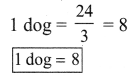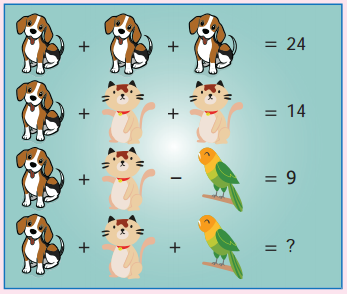(ii) 1 dog + 1 cat + 1 cat = 14
1 dog + 2cat = 14
8 + 2cat = 14
2cat = 14 – 8
2 cat = 6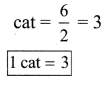ditional Questions 63″ width=”107″ height=”87″ />

(iii) 1 dog + 1 cat – 1 parrot = 9
8 + 3 – 1 parrot = 9
8 + 3 – 9 = 1 parrot
11 – 9 = 1 parrot
2 = 1 parrot
1 parrot = 2

(iv) 1 dog + 1 cat + 1 parrot = ?
8 + 3 + 2 = 13
Verification:
(i) 8 + 8 + 8 = 24
(ii) 8 + 3 + 3 = 14
(iii) 8+ 3 – 2 = 9
(iv) 8 + 3 + 2 = 13

Try These (Text book Page No. 68)

Question 1.
Kandhan and kaviya are friends. Both of them are having some pen. Kandhan: If you give me one pen then, we will have equal number of pens. Will you? Kaviya: But, if you give me one of your pens, then mine will become twice as yours. Will you?
Construct algebraic equations for this situation, can you guess and find the actual number of pens, they have?
Solution:
Let the number of pens initially Kandhan and Kaviya had be x and y respectively.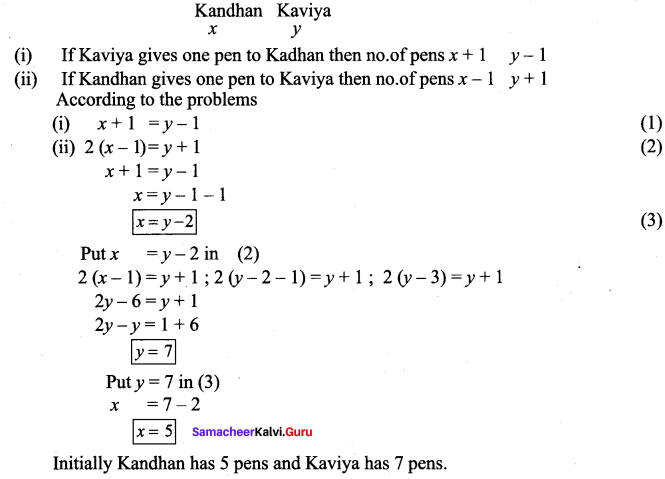## How to Prepare using Samacheer Kalvi 7th Maths Algebra Notes PDF?

Students must prepare for the upcoming exams from Samacheer Kalvi 7th Maths Algebra Notes PDF by following certain essential steps which are provided below.

• Use Samacheer Kalvi 7th Maths Algebra notes by paying attention to facts and ideas.
• Pay attention to the important topics
• Refer TN Board books as well as the books recommended.
• Correctly follow the notes to reduce the number of questions being answered in the exam incorrectly
• Highlight and explain the concepts in details.

## Frequently Asked Questions on Samacheer Kalvi 7th Maths Algebra Notes

#### How to use Samacheer Kalvi 7th Maths Algebra Notes for preparation??

Read TN Board thoroughly, make separate notes for points you forget, formulae, reactions, diagrams. Highlight important points in the book itself and make use of the space provided in the margin to jot down other important points on the same topic from different sources.

#### How to make notes for Samacheer Kalvi 7th Maths Algebra exam?

Read from hand-made notes prepared after understanding concepts, refrain from replicating from the textbook. Use highlighters for important points. Revise from these notes regularly and formulate your own tricks, shortcuts and mnemonics, mappings etc.
Share: